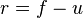# Rank correlation facts for kids

Kids Encyclopedia Facts

A rank correlation is any statistic that measures the relationship between rankings. A "ranking" is the assignment of "first", "second", "third", etc. to different observations of a variable. A rank correlation coefficient measures the degree of similarity between two rankings.

One might test for do colleges with a higher-ranked basketball program tend to have a higher-ranked football program? A quite important question is do people with higher-ranked education tend to get higher levels of income?

Some of the most used rank correlation statistics are

1. Spearman's ρ
2. Kendall's τ
3. Goodman and Kruskal's γ

An increasing rank correlation coefficient implies increasing agreement between rankings. The coefficient is inside the interval [−1, 1] and assumes the value:

• 1 if the agreement between the two rankings is perfect; the two rankings are the same.
• 0 if the rankings are completely independent.
• −1 if the disagreement between the two rankings is perfect; one ranking is the reverse of the other.

## Kerby simple difference formula

Dave Kerby recommended the rank-biserial as the measure to introduce students to rank correlation, because the general logic can be explained at an introductory level.

Kerby showed that this rank correlation can be expressed in terms of two figures: the percent of data that support a stated hypothesis, and the percent of data that do not support it. The Kerby simple difference formula states that the rank correlation can be expressed as the difference between the proportion of favorable evidence (f) minus the proportion of unfavorable evidence (u).$r = f - u$

### An illustration

Suppose a coach trains long-distance runners for one month using two methods. Group A has 5 runners, and Group B has 4 runners. The hypothesis is that method A produces faster runners. The race to assess the results finds that the runners from Group A do indeed run faster, with the following ranks: 1, 2, 3, 4, and 6. The slower runners from Group B thus have ranks of 5, 7, 8, and 9.

The analysis is done on pairs, a member of one group compared to a member of the other group. For example, the fastest runner in the study is a member of four pairs: (1,5), (1,7), (1,8), and (1,9). All four of these pairs support the hypothesis, because in each pair the runner from Group A is faster than the runner from Group B. There are a total of 20 pairs, and 19 pairs support the hypothesis. The only pair which does not support the hypothesis are the two runners with ranks 5 and 6, because in this pair, the runner from Group B had the faster time. By the Kerby simple difference formula, 95% of the data support the hypothesis (19 of 20 pairs), and 5% do not support (1 of 20 pairs), so the rank correlation is r = .95 - .05 = .90.

The maximum value for the correlation is r = 1, which means that 100% of the pairs favor the hypothesis. A correlation of r = 0 indicates that half the pairs favor the hypothesis and half do not. An effect size of r = 0 describes no relationship between group membership and the members' ranks.Rank correlation Facts for Kids. Kiddle Encyclopedia.